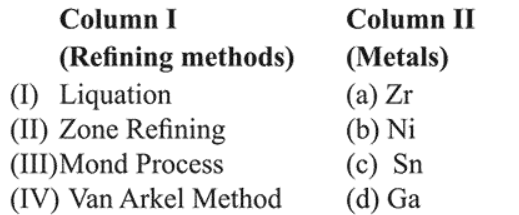# Match the refining methods (Column I) with metals (Column II).Question:

Match the refining methods (Column I) with metals (Column II).1. (I) - (c); (II) - (a); (III) - (b); (IV) - (d)

2. (I) - (b); (II) - (c); (III) - (d); (IV) - (a)

3. (I) - (c); (II) - (d); (III) - (b); (IV) - (a)

4. (I) - (b); (II) - (d); (III) - (a)I (IV) - (c)

Correct Option: , 3

Solution:

Liquation is used for $\mathrm{Sn}$, zone refining is used for $\mathrm{Ga}$, mond's process is used for refining of Ni and van Arkel method is used for $\mathrm{Zr}$, So, correct match is

(I) - (c); (II)-(d); (III)-(b); (IV)-(a)